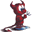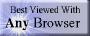music# multiplication

## summary

This subchapter looks at multiplication.

## free computer programming text book project

If you like the idea of this project,### stub section

This subchapter is a stub section. It will be filled in with instructional material later. For now it serves the purpose of a place holder for the order of instruction.

Professors are invited to give feedback on both the proposed contents and the propsed order of this text book. Send commentary to Milo, PO Box 1361, Tustin, California, 92781, USA.

# multiplication

This subchapter looks at multiplication.

### number systems

Here is a little bit of college level work on multiplication.

A system of numbers os considered to be closed under an operation if it reproduces itself.

S is a set of numbers. S is closed under multiplication if for any two numbers a and b in the set S, the product a · b is also a number in the set S. It follows that any product of two, three, four, or any finite number of elements in S also belong to S. A set closed under multiplication is called a ray.

The set of all natural numbers {1, 2, 3, …} is closed under multiplication.

The set of all integers {0, ±1, ±2, ±3, …} is closed under multiplication.

The set of all even integers {0, ±2, ±4, ±6, …} is closed under multiplication.

The set of all odd integers {±1, ±3, ±5, ±7, …} is closed under multiplication.

The set of all rational numbers is closed under multiplication.

The set of all real numbers is closed under multiplication.

The set of all complex numbers is closed under multiplication.

The set of all real numbers between zero (0) and one (1) is closed under multiplication.

A system of numbers that is closed under addition, subtraction, and multiplication is called a ring. Because all systems closed under subtraction are also closed under addition, a ring can be defined as a modul that is closed under multiplication.

Examples of rings are the set of all integers, the set of all even numbers, the set of all rational numbers, the set of all real numbers, the set of all complex numbers, the set of all complex numbers a + ib where a and b are integral, the set of all numbers of the form a + b√2 where a and b are integers, and the set of all numbers of the form a + bD where D, a, and b are integers

### functions

PL/I    PROD(x) — Pl/I built-in function that returns the total product of all of the values of the elements in an arithmetic array x. The PROD function is always carried out in floating point arithmetic. This may create small rounding errors for commercial programmers needing dollar and cents accuracy.

### assembly language instructions

For most processors, integer arithmetic is faster than floating point arithmetic. This can be reversed in special cases such digital signal processors.

On many processors, floating point arithmetic is in an optional unit or optional coprocessor rather than being included on the main processor. This allows the manufacturer to charge less for the business machines that don’t need floating point arithmetic.

The basic four integer arithmetic operations are addition, subtraction, multiplication, and division. Arithmetic operations can be signed or unsigned (unsigned is useful for effective address computations). Some older processors don’t include hardware multiplication and division. Some processors don’t include actual multiplication or division hardware, instead looking up the answer in a massive table of results embedded in the processor.

• MUL Multiply; DEC VAX; signed multiplication of scalar quantities (8, 16, or 32 bit integer or 32, 64, or 128 bit floating point) in general purpose registers or memory, available in two operand (first operand multiplied by second operand with result replacing second operand) and three operand (first operand multiplied by second operand with result placed in third operand) (MULB2 integer multiply byte 2 operand, MULB3 integer multiply byte 3 operand, MULW2 integer multiply word 2 operand, MULW3 integer multiply word 3 operand, MULL2 integer multiply long 2 operand, MULL3 integer multiply long 3 operand) (MULF2 multiply float 2 operand, MULF3 multiply float 3 operand, MULD2 multiply double float 2 operand, MULD3 multiply double float 3 operand, MULG2 multiply G float 2 operand, MULG3 multiply G float 3 operand, MULH2 multiply H float 2 operand, MULH3 multiply H float 3 operand); clears or sets flags
• MULS.W Signed Multiply; Motorola 680x0, Motorola 68300; signed multiplication of a word (16 bits) from memory or a register by a word (16 bits) in a data register with a longword (32 bit) result stored in the entire data register; sets or clears flags
• MULS.L Signed Multiply; Motorola 680x0, Motorola 68300; signed multiplication of a longword (32 bits) from memory or a register by a longword (32 bits) in a data register with a longword (32 bit) result stored in the data register (high order 32 bits of product are discarded); sets or clears flags
• MULS.L <ea>,Dh:Dl Signed Multiply; Motorola 680x0, Motorola 68300; signed multiplication of a longword (32 bits) from a data register by a longword (32 bits) in a data register with a quadword (64 bit) result stored in the data registers (high order 32 bits of product in first register, low order 32 bits of product in second data register); sets or clears flags
• MULU.W Unsigned Multiply; Motorola 680x0, Motorola 68300; unsigned multiplication of a word (16 bits) from memory or a register by a word (16 bits) in a data register with a longword (32 bit) result stored in the entire data register; sets or clears flags
• MULU.L Unsigned Multiply; Motorola 680x0, Motorola 68300; unsigned multiplication of a longword (32 bits) from memory or a register by a longword (32 bits) in a data register with a longword (32 bit) result stored in the data register (high order 32 bits of product are discarded); sets or clears flags
• MULU.L <ea>,Dh:Dl Unsigned Multiply; Motorola 680x0, Motorola 68300; unsigned multiplication of a longword (32 bits) from a data register by a longword (32 bits) in a data register with a quadword (64 bit) result stored in the data registers (high order 32 bits of product in first register, low order 32 bits of product in second data register); sets or clears flags
• MUL Unsigned Multiplication of AL or AX; Intel 80x86; unsigned multiplication of a byte (8 bits) from register or memory by the contents of the AL register with a word (16-bit) result in AX register, or unsigned multiplication of a word (16 bits) from register or memory by the contents of the AX register with a doubleword (32-bit) result in DX:AX register pair, or unsigned multiplication of a doubleword (32 bits) from register or memory by the contents of the EAX register with a quadword (64-bit) result in EDX:EAX register pair; uses an early out algorithm to speed up computations when possible; sets or clears flags
• IMUL Signed Integer Multiply; Intel 80x86; signed multiplication of a byte (8 bits), word (16 bits), or doubleword (32 bits) from register or memory by the contents of the EAX and EDX registers with result stored in the EAX and EDX registers, signed multiplication of a byte (8 bits), word (16 bits), or doubleword (32 bits) from register or memory by the contents of a register with truncated results (to size as operands) stored in the register, or signed multiplication of a byte (8 bits), word (16 bits), or doubleword (32 bits) from register or memory by the contents of an immediate value with truncated results (to size as operands) stored in any general register; uses an early out algorithm to speed up computations when possible; sets or clears flags
• MUL Multiply; MIX; multiply word or partial word field contents of memory to A-register (accumulator) with results stored in X-register and A-register pair, overflow toggle possibly set
• MR Multiply Register; IBM 360/370; RR format; signed multiply of the contents of an even numbered general purpose register (32 bits) by the contents of the immediately following odd numbered general purpose register (32 bits) with a 64-bit product in the register pair; register to register only; does not affect condition code
• M Multiply; IBM 360/370; RX format; signed multiply of the contents of a memory location (32 bits) by the contents of an odd numbered general purpose register (32 bits) with a 64-bit product in the register pair; main storage to register only; does not affect condition code
• MH Multiply Half-word; IBM 360/370; RX format; signed multiply of the contents of a memory location (16 bits) by the contents of a general purpose register (32 bits) with a 32-bit product (the high 8 bits of the true 48-bit product are discarded) in the register; main storage to register only; does not affect condition code
• EMUL Extended Multiply; DEC VAX; extended precision multiplication on operands in registers or memory, the first (longword) operand (multiplicand) is multiplied by the second (longword) operand (multiplier) giving a (doubleword) intermediary result which is stored in the fourth (doubleword) operand (product); clears or sets flags
• EMOD Extended Multiply and Integerize; DEC VAX; performs accurate range reduction of math function arguments, the floating point multiplier extension operand (second operand) is concatenated with the floating point multiplier (first operand) to gain eight additional low order fraction bits, the multiplicand operand (third operand) is multiplied by the extended multiplier operand, after multiplication the integer portion (fourth operand) is extracted and a 32 bit (EMODF) or 64 bit (EMODD) floating point number is formed from the fractional part of the product by truncating extra bits, the multiplication is such that the result is equivalent to the exact product truncated (before normalization) to a fraction field of 32 bits in floating or 64 bits in double (fifth operand); clears or sets flags

# free music player coding example

Coding example: I am making heavily documented and explained open source code for a method to play music for free — almost any song, no subscription fees, no download costs, no advertisements, all completely legal. This is done by building a front-end to YouTube (which checks the copyright permissions for you).

View music player in action: www.musicinpublic.com/.

Create your own copy from the original source code/ (presented for learning programming).

## view text bookHTML file

Because I no longer have the computer and software to make PDFs, the book is available as an HTML file, which you can convert into a PDF.

 Tweets by @osdata

## free computer programming text book project

Building a free downloadable text book on computer programming for university, college, community college, and high school classes in computer programming.

If you like the idea of this project,

send donations to:
Milo
PO Box 1361
Tustin, California 92781

Supporting the entire project:

If you have a business or organization that can support the entire cost of this project, please contact Pr Ntr Kmt (my church)

Some or all of the material on this web page appears in theThis web site handcrafted on Macintoshcomputers using Tom Bender’s Tex-Edit Plusand served using FreeBSD.†UNIX used as a generic term unless specifically used as a trademark (such as in the phrase “UNIX certified”). UNIX is a registered trademark in the United States and other countries, licensed exclusively through X/Open Company Ltd.

Names and logos of various OSs are trademarks of their respective owners.